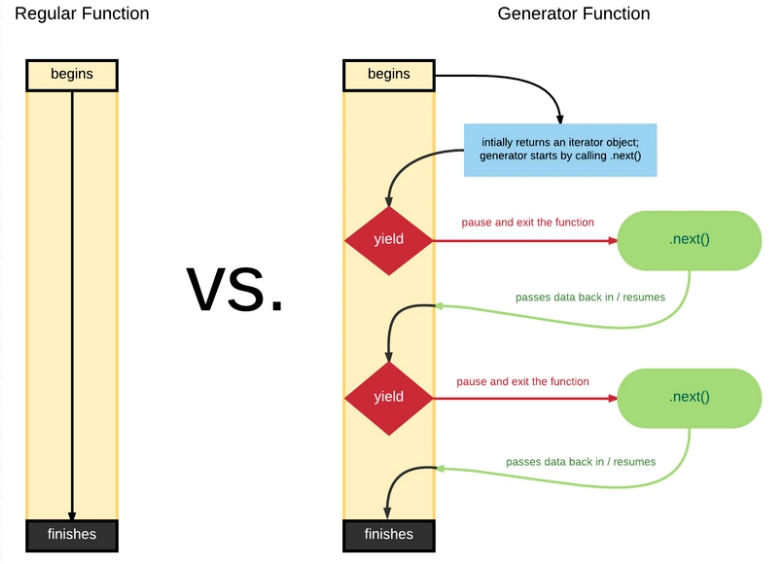# ES6系列之Generator

## 一、什么是Generator 函数

### 1.1 语法

• function* 用来声明一个函数是生成器函数，它比普通的函数声明多了一个*,*的位置比较随意可以挨着 function 关键字，也可以挨着函数名

• yield 产出的意思，这个关键字只能出现在生成器函数体内，但是生成器中也可以没有yield 关键字，函数遇到 yield 的时候会暂停，并把 yield 后面的表达式结果抛出去

• next作用是将代码的控制权交还给生成器函数

```// 声明生成器函数
function* generator() {
// A
yield 'foo'
// B
}
// 获取生成器对象
let g = generator();
// 第一个 next()，首次启动生成器
g.next(); // {value: "foo", done: false}
// 唤醒被 yield 暂停的状态
g.next();
// {value: undefined, done: true}```

### 1.2 过程分析

```// 分析一个简单例子
function* helloGenerator() {
yield "hello";
yield "generator";
return;
}
var h = helloGenerator();
console.log(h.next());//{ value: 'hello', done: false }
console.log(h.next());//{ value: 'generator', done: false }
console.log(h.next());//{ value: 'undefined', done: true }```

• 创建了h对象，指向helloGenerator的句柄

• 第一次调用next()，执行到"yield hello"，暂缓执行,并返回了"hello"

• 第二次调用next()，继续上一次的执行，执行到"yield generator",暂缓执行，并返回了"generator"。

• 第三次调用next(),直接执行return，并返回done:true，表明结束### 1.3 yield 表达式

yield是Generator函数的暂缓执行的标识，对于yield只能配合Generator函数使用，在普通的函数中使用会报错

Generator函数中还有一种yield*这个表达方式

```function* foo(){
yield "a";
yield "b";
}
function* gen(x,y){
yield 1;
yield 2;
yield* foo();
yield 3;
}
var g = gen();
console.log(g.next());//{value: 1, done: false}
console.log(g.next());//{value: 2, done: false}
console.log(g.next());//{value: "a", done: true}
console.log(g.next());//{value: "b", done: true}
console.log(g.next());//{value: "3", done: true
}```

```function* foo(){
yield "a";
yield "b";
}
function* gen(x,y){
yield 1;
yield 2;

for(var value of foo()){
yield value;
}

yield 3;
}```

## 二、Generator应用场景

### 2.1 异步操作的同步化表达

Generator函数的暂停执行的效果，意味着可以把异步操作写在yield表达式里面，等到调用next方法时再往后执行。这实际上等同于不需要写回调函数了，因为异步操作的后续操作可以放在yield表达式下面，反正要等到调用next方法时再执行。所以，Generator函数的一个重要实际意义就是用来处理异步操作，改写回调函数

```function* loadUI() {
}
// 加载UI
// 卸载UI

• 通过Generator函数部署Ajax操作，可以用同步的方式表达。

```function* main() {
var result = yield request("http://some.url");
var resp = JSON.parse(result);
console.log(resp.value);
}
function request(url) {
makeAjaxCall(url, function(response){
it.next(response);
});
}
var it = main();
it.next();```

### 2.2 控制流管理

```// 异步函数
function getDataAsync (url) {
return new Promise((resolve, reject) => {
setTimeout(() => {
var res = {
url: url,
data: Math.random()
}
resolve(res)
}, 1000)
})
}```

• 博文量
26
• 访问量
10214